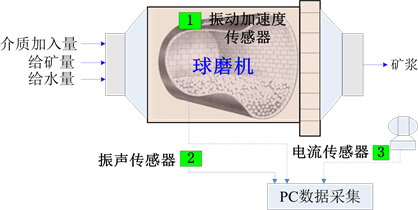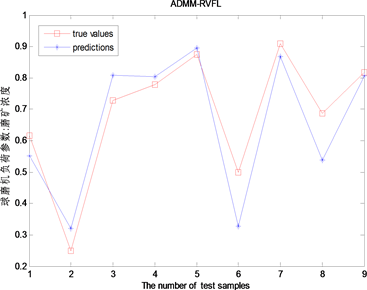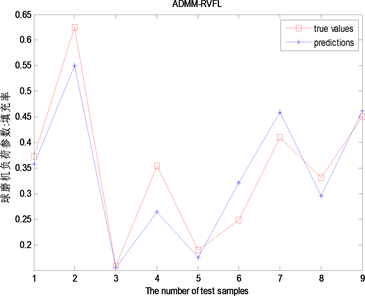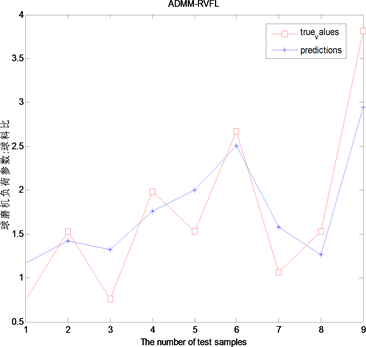# 基于交替方向乘子法的球磨机负荷分布式随机权值神经网络模型Distributed Random Weight Neural Network Model for Ball Mill Load Based on Alternating Direction Multiplier Method

• 全文下载: PDF(668KB)    PP.1-8   DOI: 10.12677/HJDM.2018.81001
• 下载量: 740  浏览量: 2,134   国家自然科学基金支持

When the traditional centralized machine learning algorithms deal with the large-scale data, there exists high communication overhead, low computational efficiency and large space complexity. A distributed random weights neural network modeling method is used to build ball mill load model based on Alternate Direction Multiplier Method (ADMM). Local network nodes are built using Random Vector Functional-Link (RVFL) network with regularized random weights, and the parameters of global distributed ball mill load model are optimized iteratively to update the solution by using the ADMM method. The experimental results show that the ADMM-RVFL-based ball mill load model has comparative advantages in terms of speed and accuracy.

1. 引言

2. 预备知识

$\begin{array}{l}\underset{u,v}{\mathrm{min}}f\left(u\right)+g\left(v\right)\\ \text{s}\text{.t}\text{.}\text{\hspace{0.17em}}Au+Bv=c\end{array}$ (1)

${\text{L}}_{P}\left(u,v,\lambda \right)=f\left(u\right)+g\left(v\right)+{\lambda }^{\text{T}}\left(Au+Bv-c\right)+\frac{\rho }{2}{‖Au+Bv-c‖}_{2}^{2}$ (2)

${u}_{k+1}:=\underset{u}{\mathrm{arg}\mathrm{min}}{\text{L}}_{P}\left(u,{v}_{k},{\lambda }_{k}\right)$ (3)

${v}_{k+1}:=\underset{v}{\mathrm{arg}\mathrm{min}}{\text{L}}_{P}\left({u}_{k+1},v,{\lambda }_{k}\right)$ (4)

${\lambda }_{k+1}:={\lambda }_{k}+\rho \left(A{u}_{k+1}+B{v}_{k+1}-c\right)$ (5)

ADMM算法类似于增广拉格朗日乘子法，实际上，也可以通过采用增广拉格朗日乘子法进行迭代求解 $u,v,\lambda$ 。如果已知道 $k$ 时刻 $\left({u}_{k},{v}_{k},{\lambda }_{k}\right)$ 数值大小，则求得：

$\left({u}_{k+1},{v}_{k+1}\right)：=\underset{u,v}{\mathrm{arg}\mathrm{min}}{\text{L}}_{P}\left(u,v,{\lambda }_{k}\right)$ (6)

${\lambda }_{k+1}:={\lambda }_{k}+\rho \left(A{u}_{k+1}+B{v}_{k+1}-c\right)$ (7)

2.2. RVFL神经网络模型

$f\left(x\right)={\sum }_{m=1}^{L}{\beta }_{m}{h}_{m}\left(x;{w}_{m}\right)={\beta }^{\text{T}}h\left(x;{w}_{1},\cdots ,{w}_{L}\right)$ (8)

$m$ 个变换通过向量 ${w}_{m}$ 进行参数化，输入是一个d维的实向量 $x\in {R}^{d}$ 。每一个 $h:x\to R$ 称为一个基，隐函数，函数链接。在仿真中使用sigmoid基础函数：

$h\left(x;w,b\right)=\frac{1}{1+\mathrm{exp}\left\{-{w}^{\text{T}}x+b\right\}}$ (9)

$H=\left(\begin{array}{ccc}{h}_{1}\left({x}_{1}\right)& \dots & {h}_{L}\left({x}_{N}\right)\\ ⋮& \ddots & ⋮\\ {h}_{1}\left({x}_{1}\right)& \dots & {h}_{L}\left({x}_{N}\right)\end{array}\right)$ (10)

${\beta }^{*}=\underset{\beta \in {R}^{L}}{\mathrm{arg}\mathrm{min}}\frac{1}{2}{‖H\beta -Y‖}_{2}^{2}+\frac{\lambda }{2}{‖\beta ‖}_{2}^{2}$ (11)

$J\left(\beta \right)$ 的梯度为零，即：

$\frac{\partial J}{\partial \beta }={H}^{\text{T}}H\beta -{H}^{\text{T}}Y+\lambda \beta =0$ (12)

${\beta }^{*}={\left({H}^{\text{T}}H+\lambda I\right)}^{-1}{H}^{\text{T}}Y$ (13)

${\left({H}^{\text{T}}H+\lambda I\right)}^{-1}{H}^{\text{T}}={H}^{\text{T}}{\left(H{H}^{\text{T}}+\lambda I\right)}^{-1}$ (14)

${\beta }^{*}={H}^{\text{T}}{\left(H{H}^{\text{T}}+\lambda I\right)}^{-1}Y$ (15)

${t}_{k}\left[n+1\right]={t}_{k}\left[n\right]+\gamma \left({\beta }_{k}\left[n+1\right]-Ζ\left[n+1\right]\right)$ (16)

${\beta }_{k}\left[n+1\right]={\left({H}_{k}^{\text{T}}{H}_{k}+\gamma Ι\right)}^{-1}\left({H}_{k}^{\text{T}}{Y}_{k}-{t}_{k}\left[n\right]+\gamma Ζ\left[n\right]\right)$ (17)

$Ζ\left[n+1\right]=\frac{\gamma \stackrel{^}{\beta }+\stackrel{^}{t}}{\frac{\lambda }{L}+\gamma }$ (18)

$\stackrel{^}{\beta }=\frac{1}{L}{\sum }_{k=1}^{L}{\beta }_{k}\left[n+1\right]$ (19)

$\stackrel{^}{t}=\frac{1}{L}{\sum }_{k=1}^{L}{t}_{k}\left[n+1\right]$ (20)

${\left({H}_{k}^{\text{T}}{H}_{k}+\gamma Ι\right)}^{-1}={\gamma }^{-1}\left[Ι-{H}_{k}^{\text{T}}\left(\gamma Ι+{H}_{k}{H}_{k}^{\text{T}}\right){H}_{k}\right]$ (21)

${r}_{k}\left[n\right]={\beta }_{k}\left[n\right]-Ζ\left[n\right]$ (22)

$s\left[n\right]=-\gamma \left(Ζ\left[n\right]-Ζ\left[n-1\right]\right)$ (23)

${‖{r}_{k}\left[n\right]‖}_{2}<{ϵ}_{\text{primal}}$ (24)

${‖s\left[n\right]‖}_{2}<{ϵ}_{\text{dual}}$ (25)

${ϵ}_{\text{primal}}=\sqrt{L}{ϵ}_{\text{abs}}+{ϵ}_{\text{rel}}\mathrm{max}\left\{{‖{\beta }_{k}\left[n\right]‖}_{2},{‖Ζ\left[n\right]‖}_{2}\right\}$ (26)

${ϵ}_{\text{dual}}=\sqrt{L}{ϵ}_{\text{abs}}+{ϵ}_{\text{rel}}{‖{t}_{k}\left[n\right]‖}_{2}$ (27)

4. 实验仿真结果与分析

4.1. 实验对象描述Figure 1. Signal acquisition of experimental ball mill

4.2. MATLAB分布式计算集群配置

4.3. 球磨机实验结果和分析

$\text{MAE}=\frac{1}{L}{\sum }_{\left({X}_{i},{y}_{i}\right)\in T}|f\left({X}_{i}\right)-{y}_{i}|$ (28)

$\text{MSE}=\frac{1}{L}{\sum }_{\left({X}_{i},{y}_{i}\right)\in T}{\left(f\left({X}_{i}\right)-{y}_{i}\right)}^{2}$ (29)

$\text{RMSE}=\frac{1}{L}\sqrt{{\sum }_{\left({X}_{i},{y}_{i}\right)\in T}{\left(f\left({X}_{i}\right)-{y}_{i}\right)}^{2}}$ (30)(a)(b)(c)Table 3. Comparison of forecasting results of filling rate with different modeling methods

5. 结论

  Zhao, L.J., Feng, X., Yuan, D.C. and Xiao, H. (2012) Feature Selection of Frequency Spectrum for the Ball Mill Load Based on Interval Partial Least Squares. Journal of Theoretical and Applied Information Technology, 43, 119-126.  Zhao, L.J., Feng, X. and Yuan, D.C. (2012) Soft Sensor Modeling of Mill Load Based on Feature Selection Using Synergy Interval PLS. Journal of Theoretical and Applied Information Technology, 44, 64-72.  Behera, B., Mishra, B.K. and Murty, C.V.R. (2007) Experimental Analysis of Charge Dynamics in Tumbling Mills by Vibration Signature Technique. Minerals Engineering, 20, 84-91. https://doi.org/10.1016/j.mineng.2006.05.007  Huang, P., Jia, M.P. and Zhong, B.L. (2009) Investigation on Measuring the Fill Level of an Industrial Ball Mill Based on the Vibration Characteristics of the Mill Shell. Minerals Engineering, 22, 1200-1208. https://doi.org/10.1016/j.mineng.2009.06.008  赵立杰, 汤健, 柴天佑. 基于选择性极限学习机集成的磨机负荷软测量[J]. 浙江大学学报(工学版), 2011, 45(12): 2088-2092.  Scardapane, S. Wang, D., Panella, M. and Uncini, A. (2015) Distributed Learning for Random Vector Functional-Link Networks. Information Sciences, 301, 271-284. https://doi.org/10.1016/j.ins.2015.01.007  Awerbuch, B. (1987) Optimal Distributed Algorithms for Minimum Weight Spanning Tree, Counting, Leader Election, and Related Problems. Proceedings of the Nineteenth Annual ACM Symposium on Theory of Computing, ACM, 230-240. https://doi.org/10.1145/28395.28421  Boyd, S., Parikh, N., Chu, E., et al. (2011) Distributed Optimization and Statistical Learning via the Alternating Direction Method of Multipliers. Foundations and Trends in Machine Learning, 3, 1-122. https://doi.org/10.1561/2200000016  Pao, Y.H., Park, G.H. and Sobajic, D.J. (1994) Learning and Generalization Characteristics of the Random Vector Functional-Link Net. Neurocomputing, 6, 163-180. https://doi.org/10.1016/0925-2312(94)90053-1